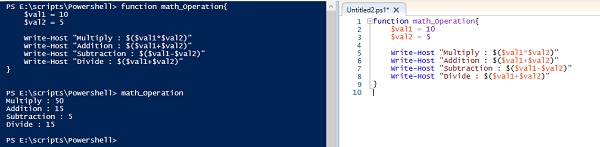# Explain the PowerShell Function.

Function in a PowerShell is to reduce the redundancy of the repeated codes this means codes which are repeating, bind them into a function and call the function whenever necessary, so you don’t need to write codes multiple times.

## Example

Let assume, you want to perform arithmetic operations (multiply, sum, divide and subtraction) of two values 5 and 4 in one go, so you will write different operations for two values or you will assign values to the variable called $val1 and$val2 and perform various operations on them as shown below in the example.

$val1 = 5$val2 = 4

$val1 *$val2
$val1 +$val2
$val1 /$val2
$val1 -$val2

Now, assume value changes again, let say 20 and 10 and change the value of the variable and need to write the whole bunch of commands again. PowerShell function plays a vital role here. So the repetitive code can be written inside the function.

Before starting our first example, we will see the syntax first.

function FunctionName(Parameter1, Parameter2,.....,ParameterN) {
//Operation commands
// Conditions
}

In the below example, we have given a function name called math_operation, which performs all the operation of the two variables. The code is given below.

## Example

function math_Operation{
$val1 = 10$val2 = 5
Write-Host "Multiply : $($val1*$val2)" Write-Host "Addition :$($val1+$val2)"
Write-Host "Subtraction : $($val1-$val2)" Write-Host "Divide :$($val1+$val2)"
}
math_Operation

To call this function, simply we can call it by its name as shown in the example.

## Output

Multiply : 50
Subtraction : 5
Divide : 15

When you write the function execute the script then you can also call the function from PowerShell console with the function name. Here, you need to write math_operation in the terminal after script execution.Now to use the function multiple times, you simply just need to pass those two values in the function so that operations between them can be performed. To do so, we use the Param block (which refers to Parameters) inside the function and declare the variables in it.

## Example

function math_Operation{
param([int]$val1,[int]$val2)
Write-Host "Multiply : $($val1*$val2)" Write-Host "Addition :$($val1+$val2)"
Write-Host "Subtraction : $($val1-$val2)" Write-Host "Divide :$($val1+$val2)"
}

Execute the above code in the PowerShell console and in the terminal itself you can see you can use two values ($val1 and$val2) as the parameters. So every time you need to pass two values to calculate the result.

## Output

PS E:\scripts\Powershell> math_Operation -val1 20 -val2 10
Multiply : 200
Divide : 30